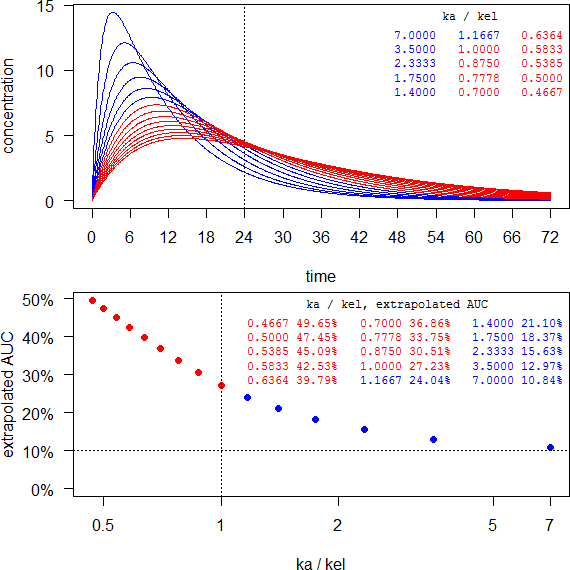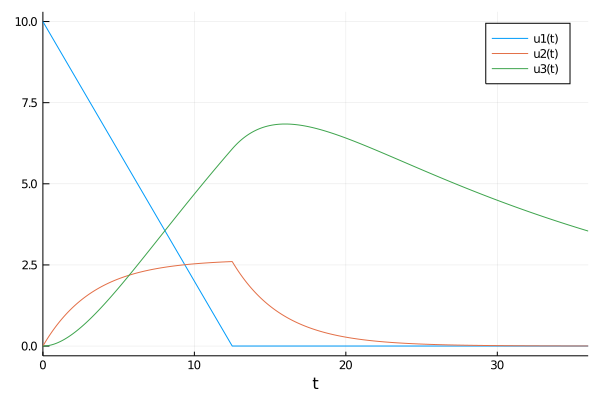Helmut
★★★Vienna, Austria,
2020-09-09 16:21
(14 d 12:06 ago)

Posting: # 21912
Views: 1,248

## EMA: Waiving MD study [Regulatives / Guidelines]

Dear all,

at the end anscript to play with.

For any elimination half life longer than ~7 hours and an intended dosing interval of 24 hours it will be impossible to get the MD study waived. Red are formulations with flip-flop PK (kakel).

last <- 72 ke   <- log(2)/7 tlag <- 0If the half life is short (say, 3 hours) you don’t have to dive deep into flip-flop PK and may succeed. However, such a limited extension might not match your development target.

last <- 36 ke   <- log(2)/3 tlag <- 1C.t <- function(f, D, V, ka, ke, t, tlag) { # 1-compartment   if (any(c(length(f) > 1, length(D) > 1, length(V) > 1,             length(ka) > 1, length(ke) > 1)))     stop("f, D, V, ka, ke have to be scalars.")   if (f <= 0 | f > 1) stop("f has to be positive <= 1.")   if (any(c(D <= 0, V <= 0, ka <= 0, ke <= 0)))     stop("D, V, ka, ke have to be positive.")   if (missing(tlag)) tlag <- 0   if (tlag < 0) stop("tlag has to be >= 0.")   if (ka != ke) {     C <- f*D*ka/(V*(ka-ke))*(exp(-ke*(t-tlag))-exp(-ka*(t-tlag)))   } else { # flip-flop PK     k <- ka     C <- f*D*k*(t-tlag)/V*exp(-k*(t-tlag))   }   C[C < 0] <- NA # negatives due to lag-time   return(C) } AUC.t <- function(C, t, tau) { # lin-up/log-down method   t <- t[t <= tau]             # for AUC until tau   AUC.t <- 0   for (j in 2:length(t)) {     if (!is.na(C[j-1])) {       if (C[j] >= C[j-1]) { # linear-up         AUC.t <- AUC.t+0.5*(t[j]-t[j-1])*(C[j]+C[j-1])       } else {              # logarithmic-down         AUC.t <- AUC.t+(t[j]-t[j-1])*(C[j]-C[j-1])/log(C[j]/C[j-1])       }     }   }   return(AUC.t) } AUC.i <- function(AUC.tau, C, t, last, tau) { # extrapolation   tlast.3  <- tail(t[t <= last], 3)   Clast.3  <- tail(C[t <= last], 3)   Clast    <- tail(Clast.3, 1)   Ctau     <- C[t == tau]   mod      <- lm(log(Clast.3) ~ tlast.3)   lambda.z <- -coef(mod)[]   if (lambda.z <= 0) stop("increasing concentrations")   AUC.inf  <- AUC.tau+Ctau/lambda.z   return(AUC.inf) } last  <- 72 tau   <- 24 f     <- 1 D     <- 100 V     <- 5 ke    <- log(2)/7       # moderate elimination half life tlag  <- 0              # optional >= 0 forms <- 15             # no. of formulations ka    <- log(2)/1:forms # vary absorption t     <- seq(0, last, 0.1) AUC.ext <- numeric() windows(width = 6, height = 6) op    <- par(no.readonly = TRUE) par(mar = c(4, 4, 0.2, 0) + 0.1, family = "sans") split.screen(c(2, 1)) screen(1)   for (j in 1:forms) {     C <- C.t(f, D, V, ka[j], ke, t, tlag)     if (j == 1) {       plot(t, C, type = "l", col = "blue", axes = FALSE,            xlab = "time", ylab = "concentration")       abline(v = tau, lty = 3)       axis(1, labels = seq(0, last, 6), at = seq(0, last, 6))       axis(2, las = 1)     } else {       if (ka[j] > ke) {         lines(t, C, col = "blue")       } else { # flip-flop         lines(t, C, col = "red")       }     }     AUC.tau    <- AUC.t(C, t, tau)     AUC.inf    <- AUC.i(AUC.tau, C, t, last, tau)     AUC.ext[j] <- (AUC.inf-AUC.tau)/AUC.inf   }   clr <- ifelse (ka/ke > 1, clr <- "blue", clr <- "red")   par(family = "mono")   legend("topright", title = "ka / kel", box.lty = 0,          legend = sprintf("%.4f", ka/ke), title.col = "black",          text.col = clr, cex = 0.75, ncol = 3); box() screen(2)   par(family = "sans")   plot(ka/ke, 100*AUC.ext, ylim = c(0, max(100*AUC.ext)), log = "x",        axes = FALSE, xlab = "ka / kel", ylab = "extrapolated AUC",        pch = 19, col = clr)   points(rev(ka/ke)[which(rev(AUC.ext) <= 0.1)],          rev(100*AUC.ext)[rev(AUC.ext) <= 0.1], pch = 21, col = "#00C000",          bg = "transparent", cex = 1.55)   xticks <- c(axTicks(side = 1, log = TRUE), max(ka/ke))   axis(1, at = xticks, labels = xticks)   axis(2, las = 1, at = c(0, pretty(100*AUC.ext)),        labels = sprintf("%.0f%%", c(0, pretty(100*AUC.ext))))   abline(v = 1, lty = 3)  # flip-flop limit   abline(h = 10, lty = 3) # MD waiver limit   par(family = "mono")   legend("topright", title = "ka / kel, extrapolated AUC", box.lty = 0,          legend = sprintf("%.4f %.2f%%", rev(ka/ke), rev(100*AUC.ext)),          title.col = "black", text.col = rev(clr), cex = 0.75, ncol = 3,          bg = "white"); box() close.screen(all = TRUE) par(op)

Dif-tor heh smusma 🖖
Helmut SchützThe quality of responses received is directly proportional to the quality of the question asked. 🚮
Science Quotes
wienui
★

Germany, Oman,
2020-09-09 17:43
(14 d 10:44 ago)

@ Helmut
Posting: # 21913
Views: 951

## EMA: Waiving MD study

Hi Helmut,

» at the end anscript to play with.
»
» For any elimination half life of > 7 hours and an intended dosing interval of 24 hours it will be impossible to get the MD study waived. Red are formulations with flip-flop PK (kakel).

Cheers,
Osama
Helmut
★★★Vienna, Austria,
2020-09-09 19:26
(14 d 09:01 ago)

@ wienui
Posting: # 21914
Views: 939

## EMA: Waiving MD study

Hi Osama,

» » at the end anscript to play with.
» »
» » For any elimination half life of > 7 hours and an intended dosing interval of 24 hours it will be impossible to get the MD study waived. Red are formulations with flip-flop PK (kakel).
»

About the script or about what I’m doing here?OK, seriously: Acc. to the EMA’s GL the MD study can be waived if AUCτ–∞ is ≤10% of AUC0–∞. Of course, in the SD study you would sample longer than τ to get a reliable estimate of λz and show that AUC0–t ≥ 80% AUC0–∞. Furthermore, you would try to show equivalence of partial AUCs.
When you develop an extended release formulation you try to slow down absorption, i.e., decrease the ka/kel-ratio – which the script does. That’s all you can do cause kel is drug-specific. In an ideal case (e.g., no absorption window) the AUC will not be affected, only Cmax will be lower and delayed. So far, so good.
But it has a nasty side effect. The early part of the AUC (until τ) will decrease and the late part increase, making waiving the MD study not possible due to the ≤10% of AUC0–∞ limit.*

Dif-tor heh smusma 🖖
Helmut SchützThe quality of responses received is directly proportional to the quality of the question asked. 🚮
Science Quotes
PharmCat
★

Russia,
2020-09-10 20:12
(13 d 08:15 ago)

@ Helmut
Posting: # 21915
Views: 846

## PK model

Hi Helmut!

I can be wrong.

I suppose when we have modified release dosage form there are can be some changes in the PK model. We shold take in to accaunt that dissolution profile of form in GI can have own dynamic. For example: if absorbtion of dissoluted drug is high and dissolution is linear we can have something with "plato" profile. So resulting PK profile can be wery surprising.
Helmut
★★★Vienna, Austria,
2020-09-10 22:26
(13 d 06:00 ago)

@ PharmCat
Posting: # 21916
Views: 838

## Zero order helps often

Hi PharmCat,

» I can be wrong.

No, you aren’t.» I suppose when we have modified release dosage form there are can be some changes in the PK model. […] So resulting PK profile can be wery surprising.

In many cases a good model is similar to an infusion (i.e., zero order input). The only difference is that instead of the length of the infusion $$t_\textrm{i}$$ the end of absorption $$t_\textrm{e}$$ is used:
$$C(t)=\frac{f\; D}{t_\textrm{e}\; V\; k_\textrm{el}}\left [\exp (k_\textrm{el}\cdot t^{*})-\exp (k_\textrm{el}\cdot t) \right ]\left\{\begin{matrix} t>t_\textrm{e} \to t^{*}=t-t_\textrm{e}\\ t\leqslant t_\textrm{e} \to t^{*}=0 \end{matrix}\right.$$Little bit tricky cause $$t_\textrm{i}$$ is a known constant and $$t_\textrm{e}$$ and estimate.

Dif-tor heh smusma 🖖
Helmut SchützThe quality of responses received is directly proportional to the quality of the question asked. 🚮
Science Quotes
PharmCat
★

Russia,
2020-09-11 02:33
(13 d 01:54 ago)

@ Helmut
Posting: # 21917
Views: 831

## Zero order helps often

Hi Helmut!

» In many cases a good model is similar to an infusion...

I thin it can be look like this:For ODE system:

 function pkf!(du,u,p,t)     if u > 0 px = 0.8 else px = 0 end     du = - px     du = - p * u + px     du =   p * u - p * u end u0 = [10.000, 0.000, 0.0] p = [0.3, 0.04] 
mittyri
★★

Russia,
2020-09-11 05:31
(12 d 22:56 ago)

@ PharmCat
Posting: # 21918
Views: 826

## Distributed delay

Hi PharmCat,

»one step further and you are on Gamma Distributed delay(Savic, Hu)

Kind regards,
MittyriIng. Helmut Schütz